# Reducing fractions, the construction of fractions to a common denominator

Using basic properties of fractions , it is possible to replace a given fraction of others equal to this, but with a smaller numerator and denominator. This substitution is called reduction of fractions.To reduce a fractionyou need to find the greatest common divisor of its numerator and denominator: GCD (m,n), and then divide the numerator and denominator by this number. If GCD (m,n)=1, the fraction cannot be shortened.

## Examples of reducing fractions

Example 1: simplify the fraction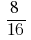Solution: GCD (8;16) = 8, then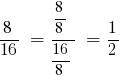Example 2: simplify the fraction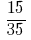Solution: GCD (5;35) = 5, then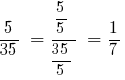Example 3: simplify the fraction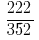Solution: GCD (222;352) = 2, thenExample 4: simplify the fraction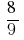Solution: GCD (8;9) = 1, then, by definition, the fraction cannot be shortened.

## The construction of fractions to a common denominator

Fractions reduce to the lowest common denominator. It is equal to the least common multiple (LCM) of the denominators of these fractions, that is, the smallest number that is divisible by the denominator of the first and second fractions.

### The construction of fractions examples

Example 1: to bring to common denominator: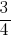and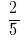NSK (4, 5) = 20.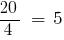— auxiliary multiplier of the first fraction,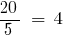— auxiliary multiplier for the second fraction.

Then: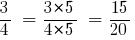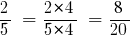Answer: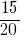andExample 2: to Reduce to a common denominator: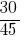andNSK (45, 15) = 45.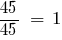— auxiliary multiplier of the first fraction,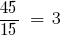— auxiliary multiplier for the second fraction.

Then: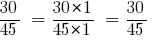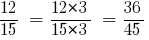Answer: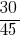and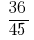Tags:
Chapter:
Versions in other languages:
Share with friends: